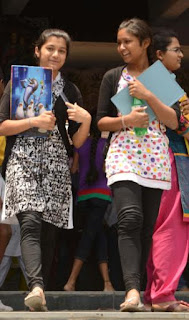## August 19, 2016

### Senior Inter Maths Part B Preparation Strategy for Telangana and AP ExamsPaper - B:  The Circle is the first chapter in 'Coordinate Geometry' unit. It is defined in terms of locus, which was studied in first year paper-B. Locus defines various geometrical figures in coordinate geometry. Also, the chapter 'Translation of Axes' is used to derive important results such as parametric equations of a circle. "Learn to be silent and let your quiet mind listen and absorb" is the famous quotation best suitable in class rooms in learning about the circle and its various features.

Certain results that were studied in first year coordinate geometry are widely used in obtaining certain other results in second year coordinate geometry. For example, the foot of the perpendicular relation studied in 'Straight Line' is used to find the midpoint of the chord intercepted by a given circle and a line. The section formulae will come into use when it is required to find the relative positions of two circles. Thus, students are expected to remember the fundamentals, studied earlier.

The second chapter 'System of Circles' deals with more than one circle. The angle between two circles and radical axis concept are well studied in this chapter. While 'Law of Cosine' formula is used in measuring the angle between two intersecting circles, the concept of locus finds its way to define radical axis of two circles. The concept of S + .L = 0, of first year straight line is applied in solving certain problems related to radial axis.

Study 'Circle' well and you can follow 'conics' as well. All the concepts of 'circle' are helpful to understand the concepts of 'conics'. The three 'conics' are very important as far as examinations are concerned. . The conic 'Hyperbola' is important by virtue of the 'Asymptotes'. 'Asymptotes' is a special feature of the 'Hyperbola'. The angle between asymptotes is an important phenomenon of hyperbola.

'Calculus' unit deals with integration mainly in the second year intermediate course. Integration is regarded as the inverse process of differentiation. Standard forms, properties of integrals, integration by parts, partial fractions method, reduction formulae etc. are all important features of integration. . Thorough understanding of integration leads to thoroughly understand 'Definite Integrals'. This chapter is very much significant as it involves various properties and formulae. Students are advised to attempt the solved and exercise problems of the text book repeatedly. The grip over integration and definite integrals helps the students to perform well in examinations at any level.

'Differential Equations' is the last chapter in Paper-B. Forming and solving the equations are the two phases of study in this chapter. Finding the order and degree of a differential equation matters most as far as very short answer type questions are concerned. 8.1.5 must be referred to by any student along with the other exercise problems. . Students must concentrate on all the methods of "solving the differential equations". Thorough and repeated practice of the exercise problems is beneficial to the students to the extent of maximum score.

Now, the tentative chapterwise weightage of marks is given below to enable the students to plan further towards getting a reasonable and respectable score in the second year papers of the subject "Mathematics".

Circles - 22
System of Circles - 6
Parabola - 9 ★ Ellipse - 8
Hyperbola - 6
Integration - 18
Definite Integrals - 15
Differential Equations - 13